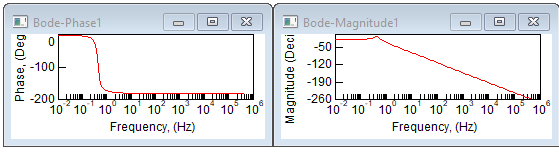### Generating Bode plots

The Frequency Response command lets you set the phase and gain margins before displaying the Bode phase and magnitude plots, like the ones shown below:Both plots are relative to log frequency (in rad/s units). The magnitude units are log and the phase units are degrees.

Before you generate Bode magnitude and phase plots of a system, prepare the system for linearization.

To generate Bode magnitude and phase plots

1.    Choose Analyze > Frequency Range to define the range and resolution of the natural

2.    Choose Analyze > Frequency Response to set the phase and gain margins and create the Bode phase and magnitude plots.